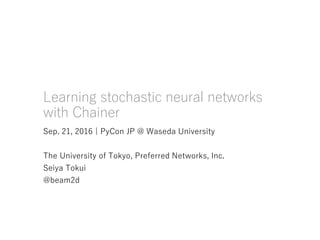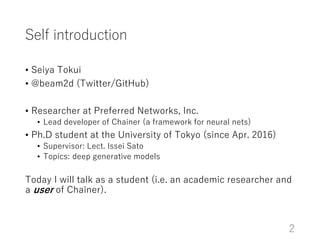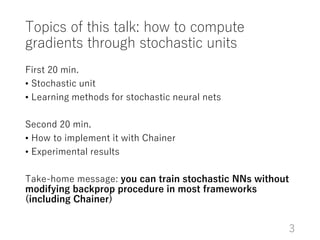Successfully reported this slideshow.

# Learning stochastic neural networks with Chainer×

# Learning stochastic neural networks with Chainer

Slides presented at PyCon JP 2016. 「確率的ニューラルネットの学習と Chainer による実装」というタイトルで PyCon JP 2016 で講演したときのスライドです。

Slides presented at PyCon JP 2016. 「確率的ニューラルネットの学習と Chainer による実装」というタイトルで PyCon JP 2016 で講演したときのスライドです。

### Learning stochastic neural networks with Chainer

1. 1. Learning stochastic neural networks with Chainer Sep. 21, 2016 | PyCon JP @ Waseda University The University of Tokyo, Preferred Networks, Inc. Seiya Tokui @beam2d
2. 2. Self introduction • Seiya Tokui • @beam2d (Twitter/GitHub) • Researcher at Preferred Networks, Inc. • Lead developer of Chainer (a framework for neural nets) • Ph.D student at the University of Tokyo (since Apr. 2016) • Supervisor: Lect. Issei Sato • Topics: deep generative models Today I will talk as a student (i.e. an academic researcher and a user of Chainer). 2
3. 3. Topics of this talk: how to compute gradients through stochastic units First 20 min. • Stochastic unit • Learning methods for stochastic neural nets Second 20 min. • How to implement it with Chainer • Experimental results Take-home message: you can train stochastic NNs without modifying backprop procedure in most frameworks (including Chainer) 3
4. 4. Caution!!! This talk DOES NOT introduce • basic maths • backprop algorithm • how to install Chainer (see the official documantes!) • basic concept and usage of Chainer (ditto!) I could not avoid using some math to explain the work, so just take this talk as an example of how a researcher writes scripts in Python. 4
5. 5. Stochastic units and their learning methods
6. 6. Neural net: directed acyclic graph of linear-nonlinear operations Linear Nonlinear 6 All operations are deterministic and differentiable
7. 7. Stochastic unit: a neuron with sampling Case 1: (diagonal) Gaussian This unit defines a random variable, and forward-prop do its sampling. 7 Linear-Nonlinear Sampling
8. 8. Stochastic unit: a neuron with sampling 8 Linear-Nonlinear Sampling (sigmoid) Case 2: Bernoulli (binary unit, taking 1 with probability )
9. 9. Applications of stochastic units Stochastic feed-forward networks • Non-deterministic prediction • Used for multi-valued predictions • E.g. inpainting lower-part of given images Learning generative models • Loss function is often written as a computational graph including stochastic units • E.g. variational autoencoder (VAE) 9
10. 10. Gradient estimation of stochastic NN is difficult! Stochastic NN is NOT deterministic -> we have to optimize expectation over the stochasticity • All possible realization of stochastic units should be considered (with losses weighted by the probability) • Enumerating all such realizations is infeasible! • We cannot enumerate all samples from Gaussian • Even in case of using Bernoulli, it costs time for units -> need approximation 10 we want to optimize it!
11. 11. General trick: likelihood-ratio method • Do forward prop with sampling • Decrease the probability of chosen values if the loss is high • difficult to decide whether the loss this time is high or low… -> decrease the probability by an amount proportional to the loss • Using log-derivative results in unbiased gradient estimate Not straight-forward to implement on NN frameworks (I’ll show later) 11 “sampled from”sampled loss log derivative
12. 12. Technique: LR with baseline LR method results in high variance • The gradient is accurate only after observing many samples (because the log-derivative is not related to the loss function) We can reduce the variance by shifting the loss value by a constant: using instead of • It does not change the relative goodness of each sample • The shift is called baseline 12
13. 13. Modern trick: reparameterization trick Write the sampling procedure as a differentiable computation • Given noise, the computation is deterministic and differentiable • Easy to implement on NN frameworks (as easy as dropout) • The variance is low!! 13 noise
14. 14. Summary of learning stochastic NNs For Gaussian units, we can use reparameterization trick • It has low variance so that we can train them efficiently For Bernoulli units, we have to use likelihood-ratio methods • It has high variance, which is problematic • In order to capture discrete nature of data representation, it is better to use discrete units, so we have to develop a fast algorithm of learning discrete units 14
15. 15. Implementing stochastic NNs with Chainer
16. 16. Task 1: variational autoencoder (VAE) Autoencoder with the hidden layer being diagonal Gaussian with reparameterization trick 16 reconstruction loss encoder decoder KL loss (regularization)
17. 17. 17 class VAE(chainer.Chain): def __init__(self, encoder, decoder): super().__init__(encoder=encoder, decoder=decoder) def __call__(self, x): mu, ln_var = self.encoder(x) # You can also write: # z = F.gaussian(mu, ln_var) sigma = F.exp(ln_var / 2) eps = self.xp.random.rand(*mu.data.shape) z = mu + sigma * eps x_hat = self.decoder(z) recon_loss = F.gaussian_nll(x, x_hat) kl_loss = F.gaussian_kl_divergence(mu, ln_var) return recon_loss + kl_loss
18. 18. 18 class VAE(chainer.Chain): def __init__(self, encoder, decoder): super().__init__(encoder=encoder, decoder=decoder) def __call__(self, x): mu, ln_var = self.encoder(x) # You can also write: # z = F.gaussian(mu, ln_var) sigma = F.exp(ln_var / 2) eps = self.xp.random.rand(*mu.data.shape) z = mu + sigma * eps x_hat = self.decoder(z) recon_loss = F.gaussian_nll(x, x_hat) kl_loss = F.gaussian_kl_divergence(mu, ln_var) return recon_loss + kl_loss stochastic part Just returning the stochastic loss. Backprop through the sampled loss estimates the gradient.
19. 19. Task 2: variational learning of sigmoid belief network (SBN) Hierarchical autoencoder with Bernoulli units 19 (2 hidden layers case) sampled loss
20. 20. 20 Parameter and forward-prop definitions class SBNBase(chainer.Chain): def __init__(self, n_x, n_z1, n_z2): super().__init__( q1=L.Linear(n_x, n_z1), # q(z_1|x) q2=L.Linear(n_z1, n_z2), # q(z_2|z_1) p1=L.Linear(n_z1, n_x), # p(x|z_1) p2=L.Linear(n_z2, n_z1), # p(z_1|z_2) ) self.add_param('prior', (1, n_z2)) # p(z_2) self.prior.data.fill(0) def bernoulli(self, mu): # sampling from Bernoulli noise = self.xp.random.rand(*mu.data.shape) return (noise < mu.data).astype('float32') def forward(self, x): a1 = self.q1(x) mu1 = F.sigmoid(a1) z1 = self.bernoulli(mu1) a2 = self.q2(z1) mu2 = F.sigmoid(a2) z2 = self.bernoulli(mu2) return (a1, mu1, z1), (a2, mu2, z2) 1st layer 2nd layer
21. 21. 21 Parameter and forward-prop definitions class SBNBase(chainer.Chain): def __init__(self, n_x, n_z1, n_z2): super().__init__( q1=L.Linear(n_x, n_z1), # q(z_1|x) q2=L.Linear(n_z1, n_z2), # q(z_2|z_1) p1=L.Linear(n_z1, n_x), # p(x|z_1) p2=L.Linear(n_z2, n_z1), # p(z_1|z_2) ) self.add_param('prior', (1, n_z2)) # p(z_2) self.prior.data.fill(0) def bernoulli(self, mu): # sampling from Bernoulli noise = self.xp.random.rand(*mu.data.shape) return (noise < mu.data).astype('float32') def forward(self, x): a1 = self.q1(x) mu1 = F.sigmoid(a1) z1 = self.bernoulli(mu1) a2 = self.q2(z1) mu2 = F.sigmoid(a2) z2 = self.bernoulli(mu2) return (a1, mu1, z1), (a2, mu2, z2) 1st layer 2nd layer initialize parameters
22. 22. 22 Parameter and forward-prop definitions class SBNBase(chainer.Chain): def __init__(self, n_x, n_z1, n_z2): super().__init__( q1=L.Linear(n_x, n_z1), # q(z_1|x) q2=L.Linear(n_z1, n_z2), # q(z_2|z_1) p1=L.Linear(n_z1, n_x), # p(x|z_1) p2=L.Linear(n_z2, n_z1), # p(z_1|z_2) ) self.add_param('prior', (1, n_z2)) # p(z_2) self.prior.data.fill(0) def bernoulli(self, mu): # sampling from Bernoulli noise = self.xp.random.rand(*mu.data.shape) return (noise < mu.data).astype('float32') def forward(self, x): a1 = self.q1(x) mu1 = F.sigmoid(a1) z1 = self.bernoulli(mu1) a2 = self.q2(z1) mu2 = F.sigmoid(a2) z2 = self.bernoulli(mu2) return (a1, mu1, z1), (a2, mu2, z2) sample through the encoder 1st layer 2nd layer
23. 23. This code computes the following loss value: bernoulli_nll(a, z) computes the following value: 23 class SBNLR(SBNBase): def expected_loss(self, x, forward_result): (a1, mu1, z1), (a2, mu2, z2) = forward_result neg_log_p = (bernoulli_nll(z2, self.prior) + bernoulli_nll(z1, self.p2(z2)) + bernoulli_nll(x, self.p1(z1))) neg_log_q = (bernoulli_nll(z1, mu1) + bernoulli_nll(z2, mu2)) return F.sum(neg_log_p - neg_log_q) def bernoulli_nll(x, y): return F.sum(F.softplus(y) - x * y, axis=1)
24. 24. How can we compute gradient through sampling? Recall likelihood-ratio: We can fake the gradient-based optimizer by passing a fake loss value whose gradient is the LR estimate 24
25. 25. How can we compute gradient through sampling? Recall likelihood-ratio: We can fake the gradient-based optimizer by passing a fake loss value whose gradient is the LR estimate 25 def __call__(self, x): forward_result = self.forward(x) loss = self.expected_loss(x, forward_result) (a1, mu1, z1), (a2, mu2, z2) = forward_result fake1 = loss.data * bernoulli_nll(z1, a1) fake2 = loss.data * bernoulli_nll(z2, a2) fake = F.sum(fake1) + F.sum(fake2) return loss + fake fake loss Optimizer runs backprop from this value
26. 26. Other note on experiments (1) Plain LR does not learn well. It always needs to use baseline. • There are many techniques, including • Moving average of the loss value • Predict the loss value from the input • Optimal constant baseline estimation Better to use momentum SGD and adaptive learning rate • = Adam • Momentum effectively reduces the gradient noise 26
27. 27. Other note on experiments (2) Use Trainer! • snapshot extension makes it easy to do resume/suspend, which is crucial for handling long experiments • Adding a custom extension is super-easy: I wrote • an extension to hold the model of the current best validation score (for early stopping) • an extension to report variance of estimated gradients • an extension to plot the learning curve at regular intervals Use report function! • It is easy to collect statistics of any values which are computed as by-products of forward computation 27
28. 28. Example of report function 28 def expected_loss(self, x, forward_result): (a1, mu1, z1), (a2, mu2, z2) = forward_result neg_log_p = (bernoulli_nll(z2, self.prior) + bernoulli_nll(z1, self.p2(z2)) + bernoulli_nll(x, self.p1(z1))) neg_log_q = (bernoulli_nll(z1, mu1) + bernoulli_nll(z2, mu2)) chainer.report({'nll_p': neg_log_p, 'nll_q': neg_log_q}, self) return F.sum(neg_log_p - neg_log_q) LogReport extension will log the average of these reported values for each interval. The values are also reported during the validation.
29. 29. My research My current research is on low-variance gradient estimate for stochastic NNs with Bernoulli units • Need extra computation, which is embarrassingly parallelizable • Theoretically guaranteed to have lower variance than LR (even vs. LR with the optimal input-dependent baseline) • Empirically shown to be faster to learn 29
30. 30. Summary • Stochastic units introduce stochasticity to neural networks (and their computational graphs) • Reparameterization trick and likelihood-ratio methods are often used for learning them • Reparameterization trick can be implemented with Chainer as a simple feed-forward network with additional noise • Likelihood-ratio methods can be implemented with Chainer using fake loss 30